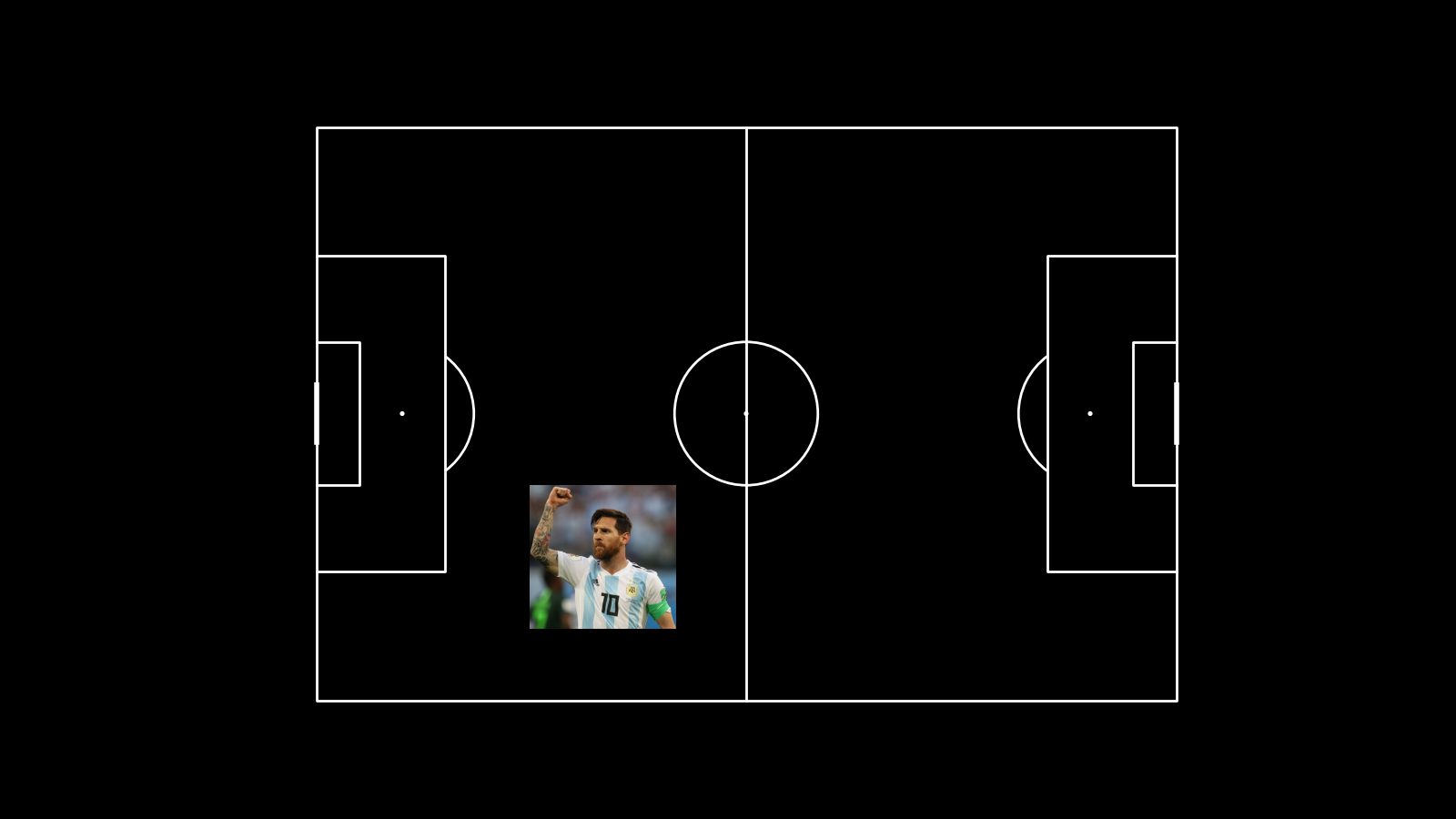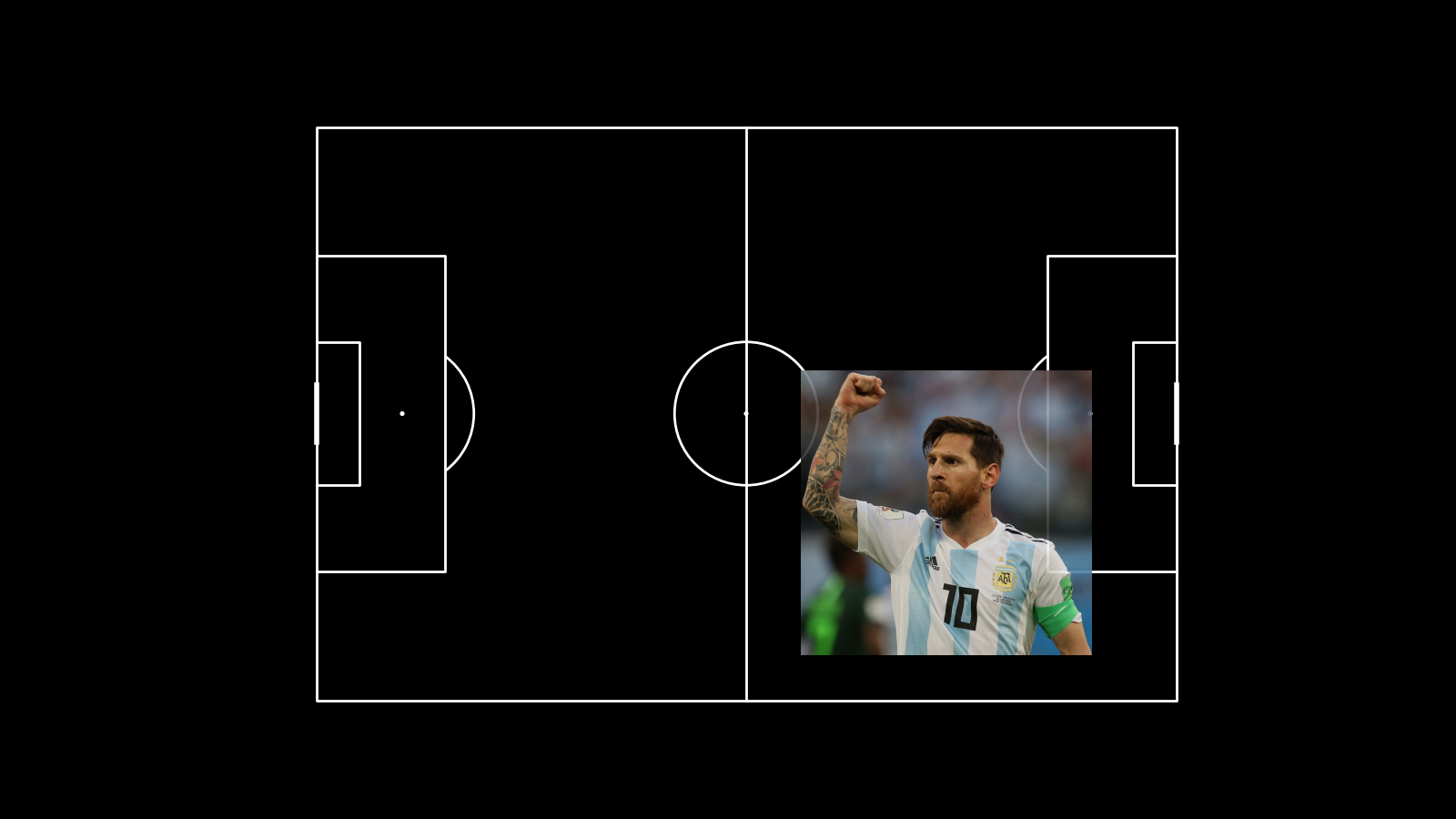# Photos

This example shows how to plot photos in your charts.

```from urllib.request import urlopen

import matplotlib.pyplot as plt
from PIL import Image

plt.style.use('dark_background')
```

## Load an image of Messi

```# load the image
image = Image.open(urlopen(IMAGE_URL))
```

## Plotting an image over a pitch

To plot images you use `Axes.imshow()` in matplotlib. We are going to draw a pitch and then overlay ontop an image of Messi on a new axis.

```# draw the pitch
pitch = Pitch(line_zorder=2)
fig, ax = pitch.draw(figsize=(16, 9), tight_layout=False)

ax_image = add_image(image, fig, left=0.55, bottom=0.2, width=0.2,
alpha=0.9, interpolation='hanning')
```## More control over the images and axis

For more control over where the images are placed, you can create a blank figure with `plt.figure()` and then use `Figure.add_axes()` to add seperate axes for each of the plot elements.

```# setup a blank figure
figsize = (16, 9)
fig_aspect = figsize / figsize
fig = plt.figure(figsize=figsize)

# setup a Pitch object

# we are going to add an axis for the pitch
# the width will be 65% (0.65) of the total figure
# we then calculate the pitch display height and draw the pitch on the new axis
PITCH_DISPLAY_WIDTH = 0.65
pitch_display_height = PITCH_DISPLAY_WIDTH / pitch.ax_aspect * fig_aspect
ax1 = fig.add_axes((0.05,  # 5% in from the left of the image
0.05,  # 5% in from the bottom of the image
PITCH_DISPLAY_WIDTH, pitch_display_height))
pitch.draw(ax=ax1, tight_layout=False)

# we are also going to add the Messi image to the top of the figure as a new axis
# but this time the width will be 8% of the figure
ax2 = add_image(image, fig, left=0.054, bottom=0.84, width=0.08, interpolation='hanning')

# and the Messi image to the bottom right of the figure on a new axis
# but this time the width will be 20% of the figure
ax3 = add_image(image, fig, left=0.75, bottom=0.054, width=0.2, interpolation='hanning')```plt.show()  # If you are using a Jupyter notebook you do not need this line